## Modeling Gas Systems

### Intended Applications

The Gas library contains basic elements, such as orifices, chambers, and pneumatic-mechanical converters, as well as sensors and sources. Use these blocks to model gas systems, for applications such as:

• Pneumatic actuation of mechanical systems

• Natural gas transport through pipe networks

• Gas turbines for power generation

• Air cooling of thermal components

You specify the gas properties in the connected loop by using the Gas Properties (G) block. This block lets you choose between three idealization levels: perfect gas, semiperfect gas, or real gas (see Gas Property Models).

Unless explicitly stated otherwise, all pressures and temperatures used in modeling gas systems are static pressure and static temperature.

### Network Variables

The Across variables are pressure and temperature, and the Through variables are mass flow rate and energy flow rate. Note that these choices result in a pseudo-bond graph, because the product of pressure and mass flow rate is not power.

### Gas Property Models

The Gas library supports perfect gas, semiperfect gas, and real gas within the same gas domain in order to cover a wide range of modeling requirements. The three gas property models provide trade-offs between simulation speed and accuracy. They also enable the incremental workflow: you start with a simple model, which requires minimal information about the working gas, and then build upon the model when more detailed gas property data becomes available.

You select the gas property model by using the Gas Properties (G) block, which specifies the gas properties in the connected circuit.

The following table summarizes the different assumptions for each gas property model.

• Thermal equation of state indicates the relationship of density with temperature and pressure.

• Caloric equation of state indicates the relationship of specific heat capacity with temperature and pressure.

• Transport properties indicate the relationship between dynamic viscosity and thermal conductivity with temperature and pressure.

Gas Property ModelThermal Equation of StateCaloric Equation of StateTransport Properties
PerfectIdeal gas lawConstantConstant
SemiperfectIdeal gas law1-D table lookup by temperature1-D table lookup by temperature
Real2-D table lookup by temperature and pressure2-D table lookup by temperature and pressure2-D table lookup by temperature and pressure

The ideal gas law is implemented in the Simscape™ Foundation Gas library as

p = ZρRT

where:

• p is the pressure.

• Z is the compressibility factor.

• R is the specific gas constant.

• T is the temperature.

The compressibility factor, Z, is typically a function of pressure and temperature. It accounts for the deviation from ideal gas behavior. The gas is ideal when Z = 1. In the perfect and semiperfect gas property models, Z must be constant but it does not have to be equal to 1. For example, if you are modeling a nonideal gas (Z ≠ 1) but the temperature and pressure of the system do not vary significantly, you can use the perfect gas model and specify an appropriate value of Z. The following table lists the compressibility factor Z for various gases at 293.15 K and 0.101325 MPa:

GasCompressibility Factor
Dry Air0.99962
Carbon Dioxide0.99467
Oxygen0.99930
Hydrogen1.00060
Helium1.00049
Methane0.99814
Natural Gas0.99797
Ammonia0.98871
R-134a0.97814

Using the perfect gas model, with the constant value of Z adjusted based on the type of gas and the operating conditions, lets you avoid the additional complexity and computational cost of moving to the semiperfect or real gas property model.

The perfect gas property model is a good starting choice when modeling a gas network because it is simple, computationally efficient, and requires limited information about the working gas. It is correct for monatomic gases and, typically, it is sufficiently accurate for gases such as dry air, carbon dioxide, oxygen, hydrogen, helium, methane, natural gas, and so on, at standard conditions.

When the gas network is operating near the saturation boundary or is operating over a very wide temperature range, the working gas can exhibit mild nonideal behavior. In this case, after successfully simulating the gas network with the perfect gas property model, consider switching to the semiperfect gas property model.

Finally, consider switching to the real gas property model if the working gas is expected to exhibit strongly nonideal behavior, such as heavy gases with large molecules. This model is the most expensive in terms of computational cost and requires detailed information about the working gas, because it uses 2-D interpolation for all properties.

### Blocks with Gas Volume

Components in the gas domain are modeled using control volumes. The control volume encompasses the gas inside the component and separates it from the surrounding environment and other components. Gas flows and heat flows across the control surface are represented by ports. The gas volume inside the component is represented using an internal node, which provides the gas pressure and temperature inside the component. This internal node is not visible, but you can access its parameters and variables using Simscape data logging. For more information, see About Simulation Data Logging.

The following blocks in the Gas library are modeled as components with a gas volume. In the case of Controlled Reservoir (G) and Reservoir (G), the volume is assumed to be infinitely large.

BlockGas Volume
Constant Volume Chamber (G)Finite
Pipe (G)Finite
Rotational Mechanical Converter (G)Finite
Translational Mechanical Converter (G)Finite
Reservoir (G)Infinite
Controlled Reservoir (G)Infinite

Other components have relatively small gas volumes, so that gas entering the component spends negligible time inside the component before exiting. These components are considered quasi-steady-state and they do not have an internal node.

### Reference Node and Grounding Rules

Unlike mechanical and electrical domains, where each topologically distinct circuit within a domain must contain at least one reference block, gas networks have different grounding rules.

Blocks with a gas volume contain an internal node, which provides the gas pressure and temperature inside the component and therefore serves as a reference node for the gas network. Each connected gas network must have at least one reference node. This means that each connected gas network must have at least one of the blocks listed in Blocks with Gas Volume. In other words, a gas network that contains no gas volume is an invalid gas network.

The Foundation Gas library contains the Absolute Reference (G) block but, unlike other domains, you do not use it for grounding gas circuits. The purpose of the Absolute Reference (G) block is to provide a reference for the Pressure & Temperature Sensor (G). However, starting in R2023a, the Pressure & Temperature Sensor (G) block contains an implicit reference node, which makes using the Absolute Reference (G) block with the Pressure & Temperature Sensor (G) block unnecessary. If you use the Absolute Reference (G) block elsewhere in a gas network, it will trigger a simulation assertion because gas pressure and temperature cannot be at absolute zero.

### Initial Conditions for Blocks with Finite Gas Volume

This section discusses the specific initialization requirements for blocks modeled with finite gas volume. These blocks are listed in Blocks with Gas Volume.

The state of the gas volume evolves dynamically based on interactions with connected blocks via mass and energy flows. The time constants depend on the compressibility and thermal capacity of the gas volume.

The state of the gas volume is represented by differential variables at the internal node of the block. As differential variables, they require initial conditions to be specified prior to the start of simulation. The dialog box of each block modeled with finite gas volume has an Initial Targets section, which lists three variables:

• Pressure of gas volume

• Temperature of gas volume

• Density of gas volume

By default, Pressure of gas volume and Temperature of gas volume have high priority, with target values equal to the standard condition (`0.101325 MPa` and ```293.15 K```). You can adjust the target values to represent the appropriate initial state of the gas volume for the block. Density of gas volume has the default priority `None` because only the initial conditions of two of the three variables are needed to completely determine the initial state of the gas volume. If desired, an alternative way to specify the initial conditions is to change Density of gas volume to high priority with an appropriate target value, and then change either Pressure of gas volume or Temperature of gas volume to a priority of none.

It is important that only two of the three variables have their priorities set to `High` for each block with a finite gas volume. Placing high-priority constraints on all three variables results in over-specification, with the solver unable to find an initialization solution that satisfies the desired initial values. Conversely, placing high-priority constraint only on one variable makes the system under-specified, and the solver might resolve the variables with arbitrary and unexpected initial values. For more information on variable initialization and dealing with over-specification, see Initialize Variables for a Mass-Spring-Damper System.

In blocks that are modeled with an infinitely large gas volume, the state of the gas volume is assumed quasisteady and there is no need to specify an initial condition.

### Choked Flow

Gas flow through Local Restriction (G), Variable Local Restriction (G), or Pipe (G) blocks can become choked. Choking occurs when the flow velocity reaches the local speed of sound. When the flow is choked, the velocity at the point of choking cannot increase any further. However, the mass flow rate can still increase if the density of the gas increases. This can be achieved, for example, by increasing the pressure upstream of the point of choking. The effect of choking on a gas network is that the mass flow rate through the branch containing the choked block depends completely on the upstream pressure and temperature. As long as the choking condition is maintained, this choked mass flow rate is independent of any changes occurring in the pressure downstream.

The following model illustrates the choked flow. In this model, the Ramp block has a slope of 0.005 and the start time of 10. The Simulink-PS Converter block has Input signal unit set to `Mpa`. All other blocks have default parameter values. Simulation time is 50 s. When you simulate the model, the pressure at port A of the Local Restriction (G) block increases linearly from atmospheric pressure, starting at 10 s. The pressure at port B is fixed at atmospheric pressure.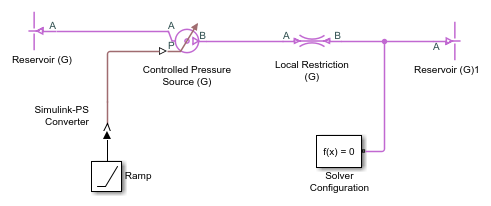The following illustration shows the logged simulation data for the Local Restriction (G) block. The Mach number at the restriction (Mach_R) reaches 1 at around 20 s, indicating that the flow is choked. The mass flow rate (mdot_A) before the flow is choked follows the typical quadratic behavior with respect to an increasing pressure difference. However, the mass flow rate after the flow is choked becomes linear because the choked mass flow rate depends only on the upstream pressure and temperature, and the upstream pressure is increasing linearly.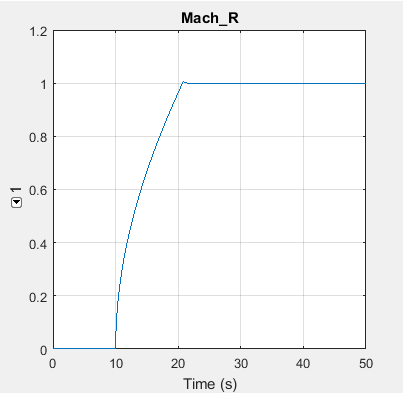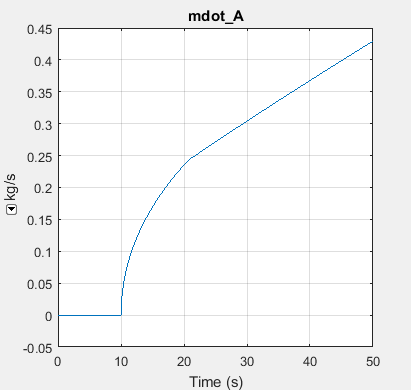The fact that the choked mass flow rate depends only on the upstream conditions can cause an incompatibility with a Mass Flow Rate Source (G) or a Controlled Mass Flow Rate Source (G) connected downstream of the choked block. Consider the model shown in the next illustration, which contains the Controlled Mass Flow Rate Source (G) block instead of the Controlled Pressure Source (G).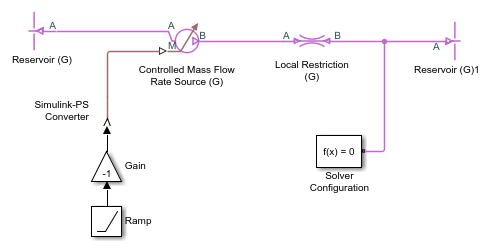If the source commanded an increasing mass flow rate from left to right through the Local Restriction (G), the simulation would succeed even if the flow became choked because the Controlled Mass Flow Rate Source (G) would be upstream of the choked block. However, in this model the Gain block reverses the flow direction, so that the Controlled Mass Flow Rate Source (G) is downstream of the choked block. The pressure upstream of the Local Restriction (G) is fixed at atmospheric pressure. Therefore, the choked mass flow rate in this situation is constant. As the commanded mass flow rate increases, eventually it will become greater than this constant value of choked mass flow rate. At this point, the commanded mass flow rate and the choked mass flow rate cannot be reconciled and the simulation fails. Viewing the logged simulation data in the Simscape Results Explorer shows that simulation fails just at the point when the Mach number reaches 1 and the flow becomes choked.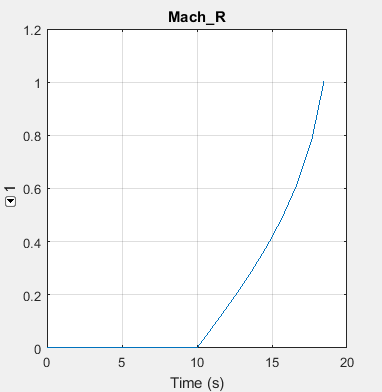In general, if a model is likely to choke, use pressure sources rather than mass flow rate sources. If a model contains mass flow rate source blocks and simulation fails, use the Simscape Results Explorer to inspect the Mach number variables in all Local Restriction (G), Variable Local Restriction (G), and Pipe (G) blocks connected along the same branch as the mass flow rate source. If the simulation failure occurs when the Mach number reaches 1, it is likely that there is a downstream mass flow rate source trying to command a mass flow rate greater than the possible choked mass flow rate.

The Mach number variable for the restriction blocks is called Mach_R. The Pipe (G) block has two Mach number variables, Mach_A and Mach_B, representing the Mach number at port A and port B, respectively.

### Flow Reversal

The flow of gas through the circuit carries energy from one gas volume to another gas volume. Therefore, the energy flow rate between two connected blocks depends on the direction of flow. If the gas flows from block A to block B, then the energy flow rate between the two blocks is based on the specific total enthalpy of block A. Conversely, if the gas flows from block B to block A, then the energy flow rate between the two blocks is based on the specific total enthalpy of block B. To smooth the transition for simulation robustness, the energy flow rate also includes a contribution based on the difference in the specific total enthalpies of the two blocks at low mass flow rates. The smoothing region is controlled by the Gas Properties (G) block parameter Mach number threshold for flow reversal.

A consequence of this approach is that the temperature of a node between two connected blocks represents the temperature of the gas volume upstream of that node. If there are two or more upstream flow paths merging at the node, then the temperature at the node represents the weighted average temperature based on the ideal mixing of the merging gas flows.

Simulation robustness can be challenging for models that exhibit quick flow reversals and large temperature differences between blocks. Quick flow reversals may be a result of having low flow resistances (for example, short pipes) between large gas volumes. Large temperature differences may be a result of the energy added by sources to maintain large pressure differences in a model with little heat dissipation. In these models, it may be necessary to increase the Mach number threshold for flow reversal parameter value to avoid simulation failure.

### Cross-Sectional Area at Block Ports

Many blocks in the gas domain let you specify the cross-sectional area at the inlet and outlet ports as a block parameter. It is recommended that you specify the same cross-sectional area for ports that are connected together. For example, if you have port A of a Constant Volume Chamber (G) block connected to a Pipe (G) block, set the Cross-sectional area at port A parameter of the Constant Volume Chamber (G) block to the same value as the Cross-sectional area parameter of the Pipe (G) block.

Especially for high-speed flows, where Mach number is close to 1, differences in the connected port areas can result in unexpected temperature differences. For more information, see Specifying the Cross-Sectional Area at Ports.